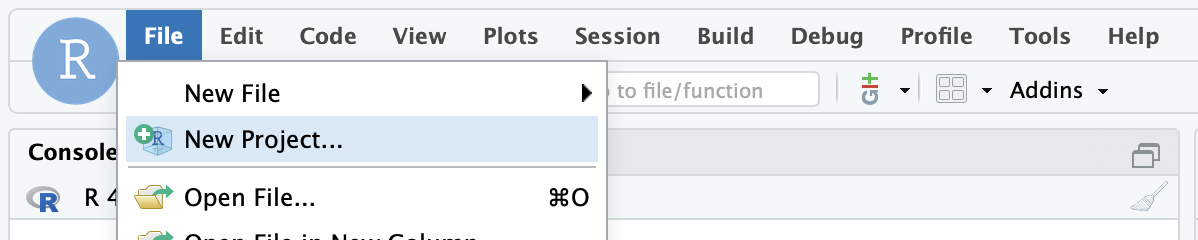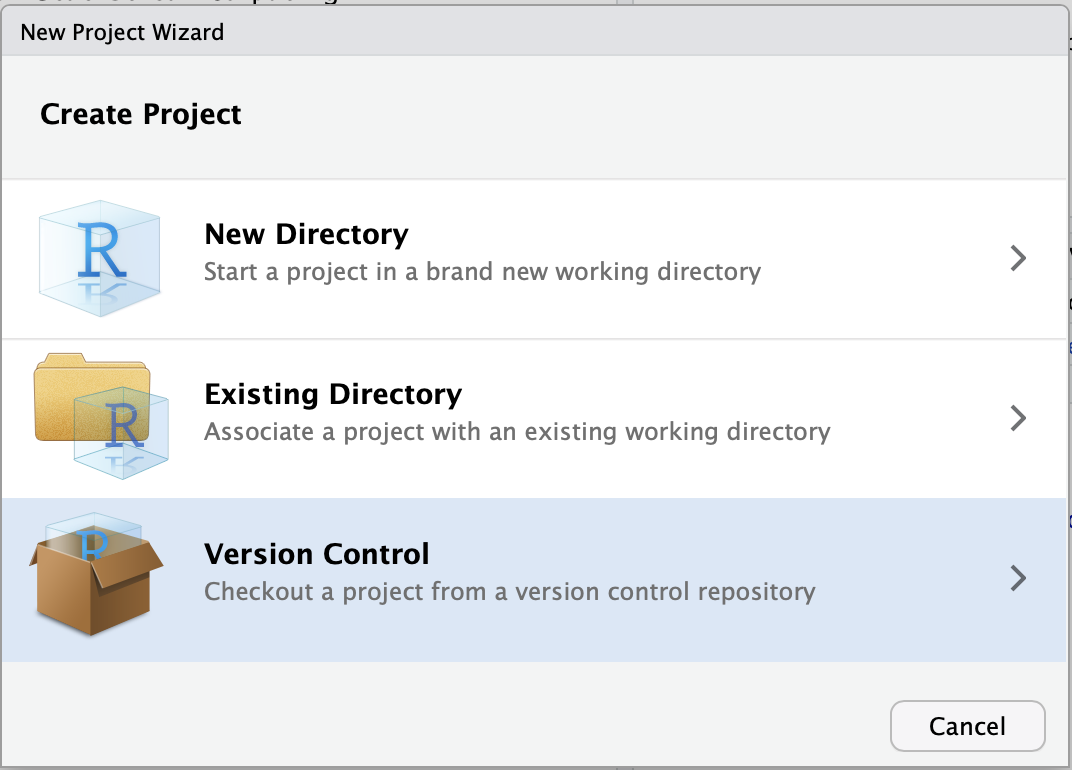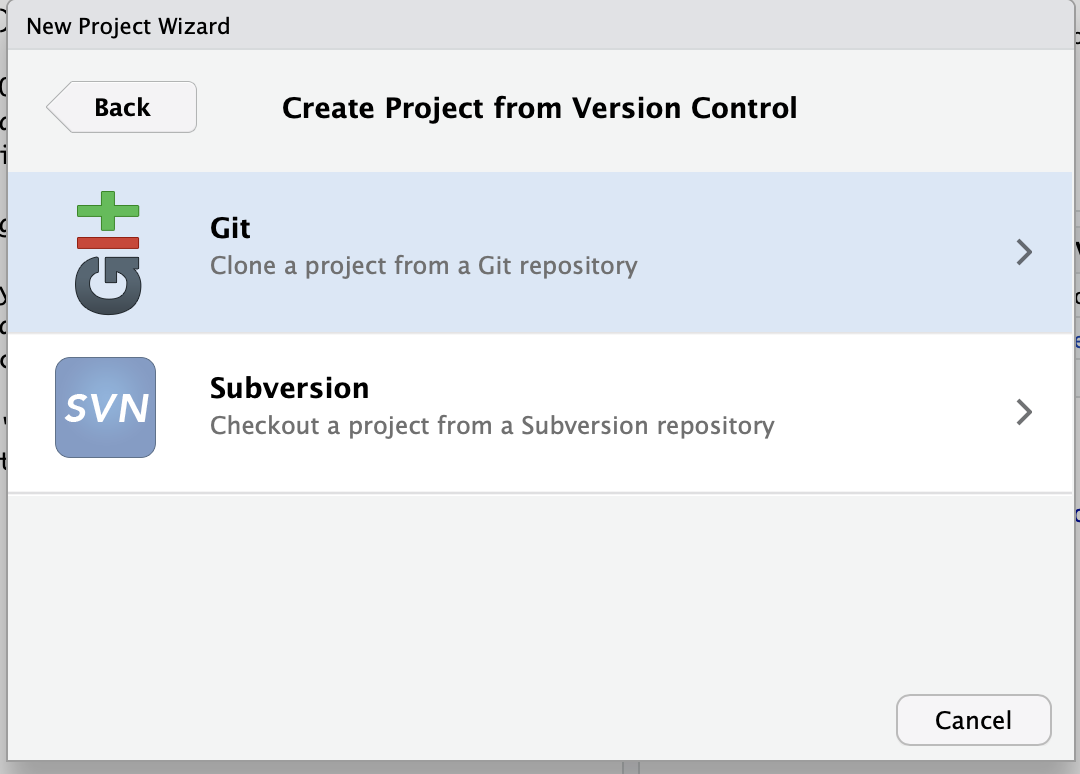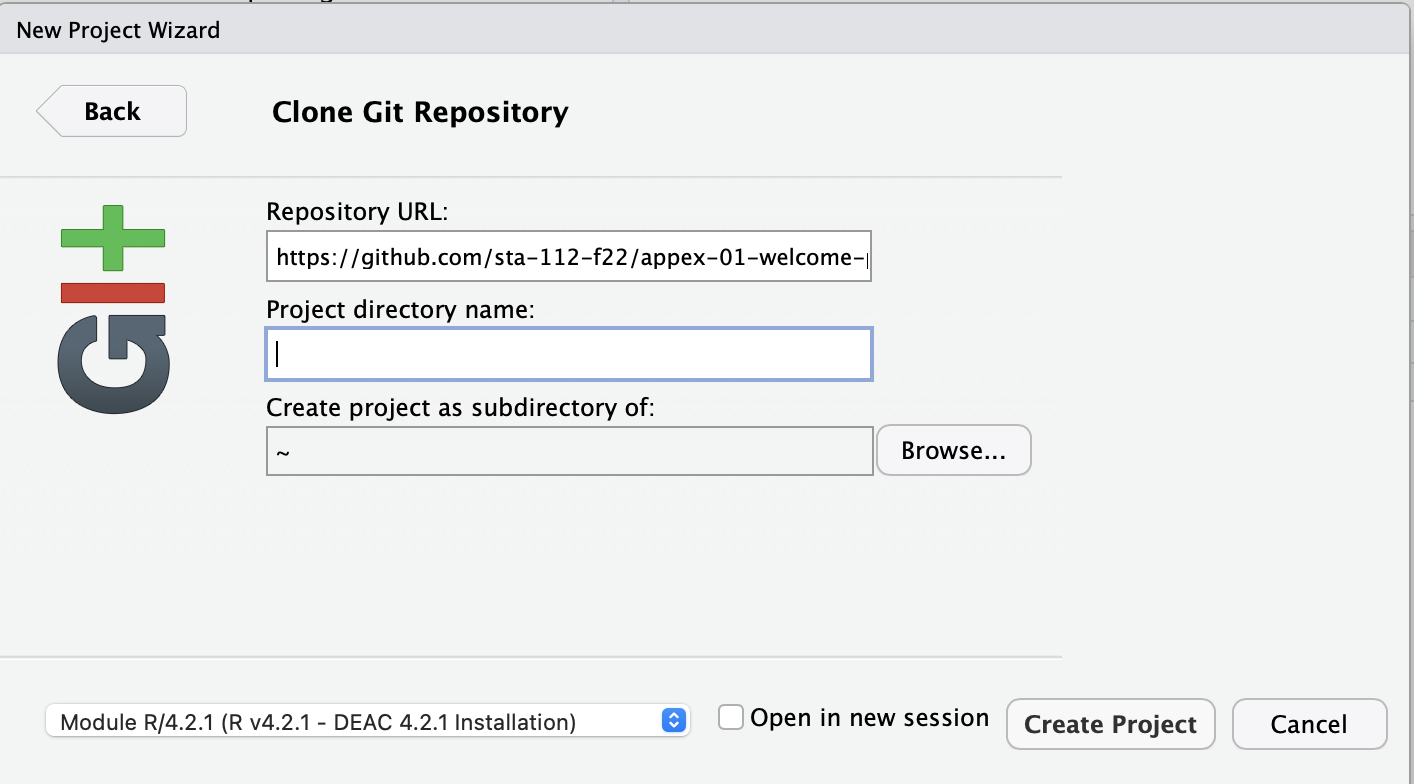# Appex 06 – tidymodels

STA 363 - Spring 2023

## Set up#### Step 1: Create a New Project

Click File > New Project#### Step 2: Click “Version Control”

Click the third option.#### Step 3: Click Git

Click the first option#### Step 4: Copy my starter files

Paste this link in the top box (Repository url):

https://github.com/sta-363-s23/06-appex.git## Part 1

1. Write a pipe that creates a model that uses lm() to fit a linear regression using tidymodels. Save it as lm_spec and look at the object. What does it return?

## Part 2

1. Fit the model:
library(ISLR)
lm_fit <- fit(lm_spec,
mpg ~ horsepower,
data = Auto)
lm_fit

Does this give the same results as

lm(mpg ~ horsepower, data = Auto)

## Part 3

1. Edit the code below to add the original data to the predicted data.
mpg_pred <- lm_fit |>
predict(new_data = Auto) |>
---

## Part 4

1. Copy the code below, fill in the blanks to fit a model on the training data then calculate the test RMSE.
set.seed(100)
Auto_split  <- ________
Auto_train  <- ________
Auto_test   <- ________
lm_fit      <- fit(lm_spec,
mpg ~ horsepower,
data = ________)
mpg_pred  <- ________ |>
predict(new_data = ________) |>
bind_cols(________)
rmse(________, truth = ________, estimate = ________)

## Part 5

1. Edit the code below to get the 5-fold cross validation error rate for the following model:

$$mpg = \beta_0 + \beta_1 horsepower + \beta_2 horsepower^2+ \epsilon$$

Auto_cv <- vfold_cv(Auto, v = 5)

results <- fit_resamples(lm_spec,
----,
resamples = ---)

results |>
collect_metrics()
• What do you think rsq is?

## Part 6

1. Fit 3 models on the data using 5 fold cross validation:

$$mpg = \beta_0 + \beta_1 horsepower + \epsilon$$

$$mpg = \beta_0 + \beta_1 horsepower + \beta_2 horsepower^2+ \epsilon$$

$$mpg = \beta_0 + \beta_1 horsepower + \beta_2 horsepower^2+ \beta_3 horsepower^3 +\epsilon$$

2. Collect the metrics from each model, saving the results as results_1, results_2, results_3

3. Which model is “best”?